# E-PolyLearning

 1. The following question consists of a set of three figures X, Y and Z showing a sequence of folding of a piece of paper. Figure (Z) shows the manner in which the folded paper has been cut. These three figures are followed by four answer figures. Choose a figure which would most closely resemble the unfolded form of Figure (Z).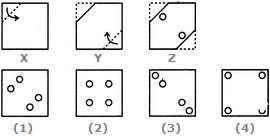a. 1 b. 2 c. 3 d. 4

 2. The following question consists of a set of three figures X, Y and Z showing a sequence of folding of a piece of paper. Figure (Z) shows the manner in which the folded paper has been cut. These three figures are followed by four answer figures. Choose a figure which would most closely resemble the unfolded form of Figure (Z).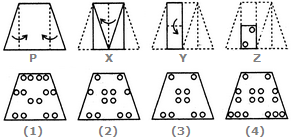a. 1 b. 2 c. 3 d. 4
 3. The following question consists of a set of three figures X, Y and Z showing a sequence of folding of a piece of paper. Figure (Z) shows the manner in which the folded paper has been cut. These three figures are followed by four answer figures. Choose a figure which would most closely resemble the unfolded form of Figure (Z).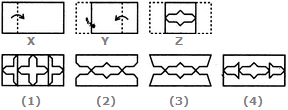a. 1 b. 2 c. 3 d. 4
 4. The following question consists of a set of three figures X, Y and Z showing a sequence of folding of a piece of paper. Figure (Z) shows the manner in which the folded paper has been cut. These three figures are followed by four answer figures. Choose a figure which would most closely resemble the unfolded form of Figure (Z).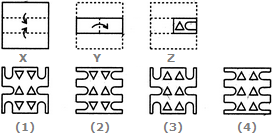a. 1 b. 2 c. 3 d. 4
 5. The following question consists of a set of three figures X, Y and Z showing a sequence of folding of a piece of paper. Figure (Z) shows the manner in which the folded paper has been cut. These three figures are followed by four answer figures. Choose a figure which would most closely resemble the unfolded form of Figure (Z).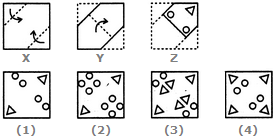a. 1 b. 2 c. 3 d. 4